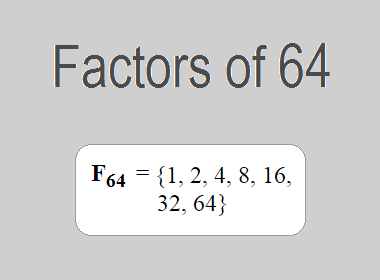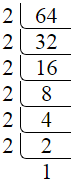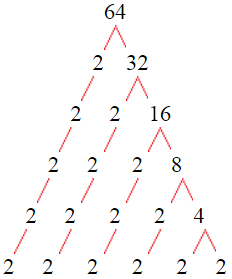# Factors of 64The factors of 64 are 1, 2, 4, 8, 16, 32, and 64 i.e. F64 = {1, 2, 4, 8, 16, 32, 64}. The factors of 64 are all the numbers that can divide 64 without leaving a remainder.

We can check if these numbers are factors of 64 by dividing 64 by each of them. If the result is a whole number, then the number is a factor of 64. Let's do this for each of the numbers listed above:

·        1 is a factor of 64 because 64 divided by 1 is 64.

·        2 is a factor of 64 because 64 divided by 2 is 32.

·        4 is a factor of 64 because 64 divided by 4 is 16.

·        8 is a factor of 64 because 64 divided by 8 is 8.

·        16 is a factor of 64 because 64 divided by 16 is 4.

·        32 is a factor of 64 because 64 divided by 32 is 2.

·        64 is a factor of 64 because 64 divided by 64 is 1.

## How to Find Factors of 64?

1 and the number itself are the factors of every number. So, 1 and 64 are two factors of 64. To find the other factors of 64, we can start by dividing 64 by the numbers between 1 and 64. If we divide 64 by 2, we get a remainder of 0. Therefore, 2 is a factor of 64. If we divide 64 by 3, we get a remainder of 1. Therefore, 3 is not a factor of 64.

Next, we can check if 4 is a factor of 64. If we divide 64 by 4, we get a remainder of 0. Therefore, 4 is also a factor of 64. We can continue this process for all the possible factors of 64.

Through this process, we can find that the factors of 64 are 1, 2, 4, 8, 16, 32, and 64. These are the only numbers that can divide 64 without leaving a remainder.

********************

********************

## Properties of the Factors of 64

The factors of 64 have some interesting properties. One of the properties is that the sum of the factors of 64 is equal to 127. We can see this by adding all the factors of 64 together:

1 + 2 + 4 + 8 + 16 + 32 + 64 = 127

Another property of the factors of 64 is that the prime factor of 64 is 2 only.

## Applications of the Factors of 64

The factors of 64 have several applications in mathematics. One of the applications is in finding the highest common factor (HCF) of two or more numbers. The HCF is the largest factor that two or more numbers have in common. For example, to find the HCF of 64 and 40, we need to find the factors of both numbers and identify the largest factor they have in common. The factors of 64 are 1, 2, 4, 8, 16, 32, and 64. The factors of 40 are 1, 2, 4, 5, 8, 10, 20, and 40. The largest factor that they have in common is 8. Therefore, the HCF of 64 and 40 is 8.

Another application of the factors of 64 is in prime factorization. Prime factorization is the process of expressing a number as the product of its prime factors. The only prime factor of 64 is 2. Therefore, we can express 64 as:

64 = 2 × 2 × 2 × 2 × 2 × 2

We can do prime factorization by division and factor tree method also. Here is the prime factorization of 64 by division method,64 = 2 × 2 × 2 × 2 × 2 × 2

Here is the prime factorization of 64 by the factor tree method,64 = 2 × 2 × 2 × 2 × 2 × 2

## Conclusion

The factors of 64 are the numbers that can divide 64 without leaving a remainder. The factors of 64 are 1, 2, 4, 8, 16, 32, and 64. The factors of 64 have some interesting properties, such as having a sum of 127. The factors of 64 have several applications in mathematics, such as finding the highest common factor and prime factorization.